PSABM5ZF - Practice---Acc7.5 Lesson 19 Fitting a Line to Data (8.SP.A.1, 8.SP.A.2)
Part A)

The scatter plot shows the number of hits and home runs for 20 baseball players who had at least 10 hits last season. The table shows the values for 15 of those players.

The model, represented by 0.15− 1.5, is graphed with a scatter plot.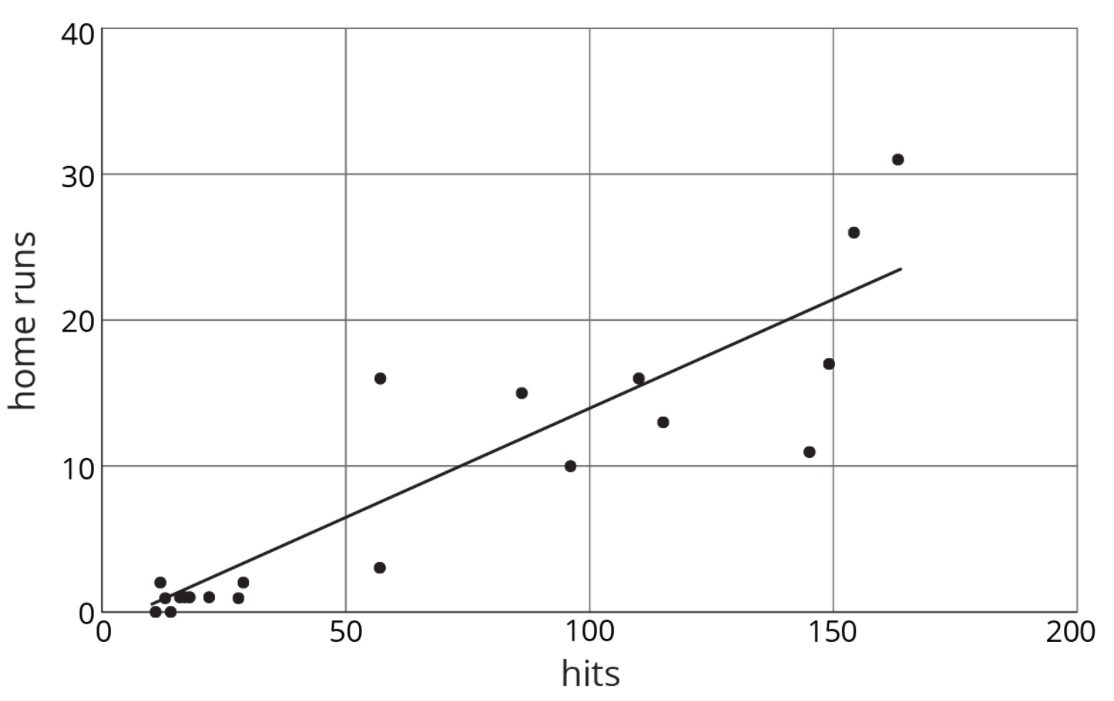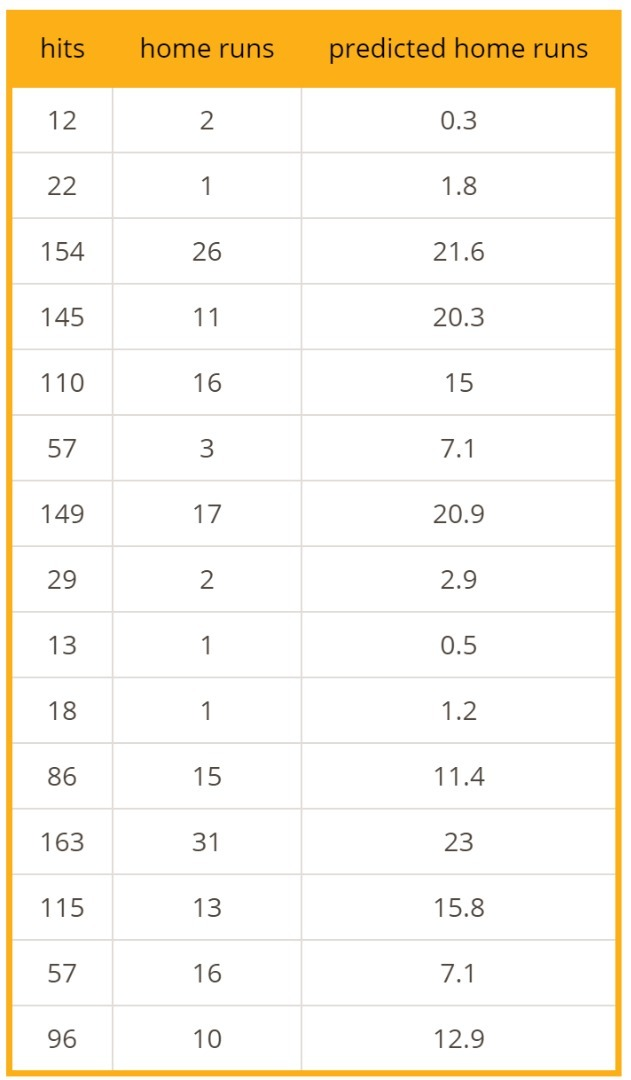Use the graph and the table to answer the questions.

Player A had 154 hits in 2015. How many home runs did he have?

Type your answer below as a number (example: 5, 3.1, 4 1/2, or 3/2):
Part B)

How many was he predicted to have?

Type your answer below as a number (example: 5, 3.1, 4 1/2, or 3/2):
Part C)

Player B was the player who most outperformed the prediction. How many hits did Player B have last season?

Type your answer below as a number (example: 5, 3.1, 4 1/2, or 3/2):
Part D)

What would you expect to see in the graph for a player who hit many fewer home runs than the model predicted?

Part A)

Here is a scatter plot that compares points per game to free throw attempts per game for basketball players in a tournament. The model, represented by y 4.413x 0.377, is graphed with the scatter plot. Here, x represents free throw attempts per game, and y represents points per game.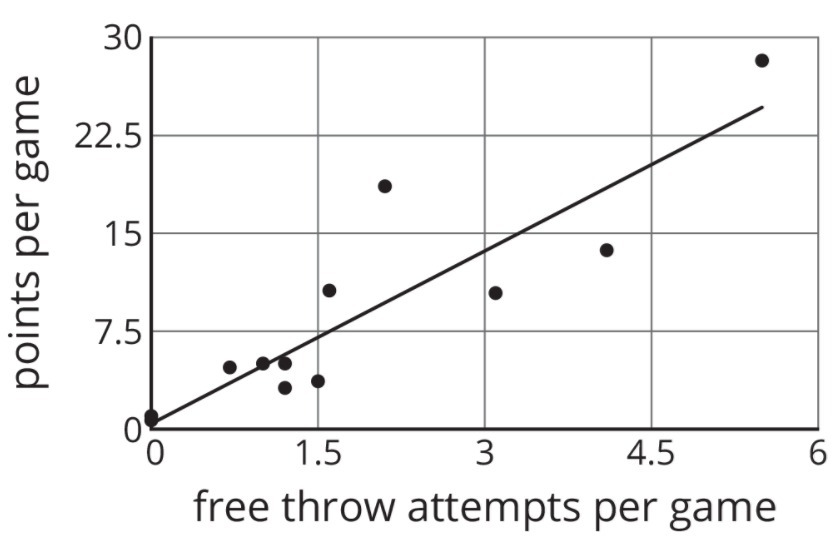Circle any data points that appear to be outliers.

Draw a graph with the circled points on paper, take a picture, and upload it using the image upload icon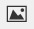.
If you do not have the ability to upload an image of your work, type "Graph is on paper."

Part B)

What does it mean for a point to be far above the line in this situation?

Part C)

Based on the model, how many points per game would you expect a player who attempts 4.5 free throws per game to have? Round your answer to the nearest tenth of a point per game.

Type your answer below as a number (example: 5, 3.1, 4 1/2, or 3/2):
Part D)

One of the players scored 13.7 points per game with 4.1 free throw attempts per game. How does this compare to what the model predicts for this player?

Choose the equation that has solutions (5,7) and (8,13).

Select one:
Part A)

Solve each equation.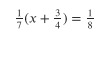x = _______

Part B)

Solve each equation.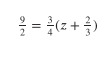z = _______

Part C)

Solve each equation.w = _______

Type your answer below as a number (example: 5, 3.1, 4 1/2, or 3/2):
Part D)

Solve each equation.a = _______